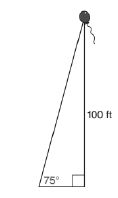Chapter 11.1, Problem 29E### Elementary Geometry for College St...

6th Edition
Daniel C. Alexander + 1 other
ISBN: 9781285195698

#### Solutions

Chapter
Section### Elementary Geometry for College St...

6th Edition
Daniel C. Alexander + 1 other
ISBN: 9781285195698
Textbook Problem
11 views

# In Exercises 27 to 34, use the drawings, where provided, to solve each problem. Angle measures should be given to the nearest degree; distances should be given to the nearest tenth of a unit.Richard sees a balloon that is 100ft above the ground. If the angle of elevation from Richard to the balloon is 75◦, how far from Richard is the balloon?To determine

To find:

The distance from Richard to balloon.

Explanation

In a right angle triangle,

1) The side PQ, which is opposite to the right angle PRQ is called the hypotenuse.

(The hypotenuse is the longest side of the right triangle.)

2) The side RQ is called the adjacent side of angle θ.

3) The side PR is called the opposite side of angle θ.

Hence, the sine ratio for an acute angle is oppositehypotenuse

Given:

From, the given diagram,

Angle measures is 75, opposite side = 100 ft.

Let a be the hypotenuse, that is the distance of the Richard from the balloon.

We know that,

sinθ=oppositeHypotenuse

sin75°=100a

From the table 11

### Still sussing out bartleby?

Check out a sample textbook solution.

See a sample solution

#### The Solution to Your Study Problems

Bartleby provides explanations to thousands of textbook problems written by our experts, many with advanced degrees!

Get Started